# arithmetic progression

## Rainbow AP(4) in an almost equinumerous coloring ★★

Author(s): Conlon

Problem   Do 4-colorings of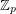, fora large prime, always contain a rainbow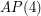if each of the color classes is of size of eitheror?

Keywords: arithmetic progression; rainbow

## Long rainbow arithmetic progressions ★★

Author(s): Fox; Jungic; Mahdian; Nesetril; Radoicic

Forlet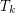denote the minimal numbersuch that there is a rainbow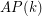in every equinumerous-coloring of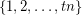for everyConjecture   For all,.

Keywords: arithmetic progression; rainbow

## Concavity of van der Waerden numbers ★★

Author(s): Landman

Forandpositive integers, the (mixed) van der Waerden numberis the least positive integersuch that every (red-blue)-coloring ofadmits either a-term red arithmetic progression or an-term blue arithmetic progression.

Conjecture   For allandwith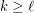,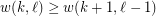.

Keywords: arithmetic progression; van der Waerden